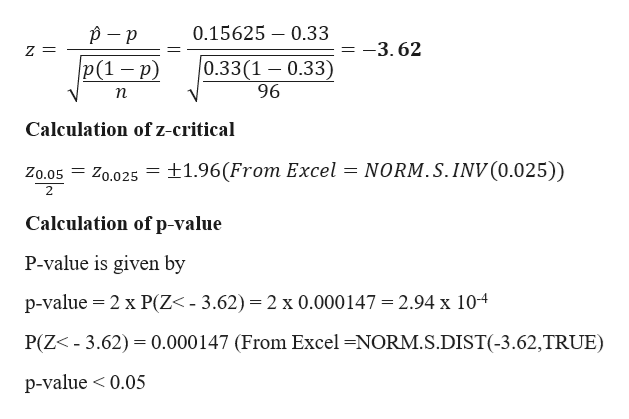# At a certain university, 16% of students fail general chemistry on their first attempt. Professor Brown teaches at this university and believes that the rate of first-time failure in his general chemistry classes is 33%. He samples 96 students from last semester who were first-time enrollees in general chemistry and finds that 15 of them failed his course.i) State the appropriate null and alternate hypotheses.ii) Compute the test statistic.iii) Using α=0.05, can you conclude that the percentage of failures differs from 33%?

Question
20 views

At a certain university, 16% of students fail general chemistry on their first attempt. Professor Brown teaches at this university and believes that the rate of first-time failure in his general chemistry classes is 33%. He samples 96 students from last semester who were first-time enrollees in general chemistry and finds that 15 of them failed his course.

i) State the appropriate null and alternate hypotheses.

ii) Compute the test statistic.

iii) Using α=0.05, can you conclude that the percentage of failures differs from 33%?

check_circle

Step 1

Given Data

Step 2

i)

Formulation of Hypotheses:

The  objective is to test, whether or not the proportion of first time failure rate is equal to 0.33

The null hypothesis is:

H0­ : p = 0.33

The alternative hypothesis is...help_outlineImage Transcriptionclose0.15625 – 0.33 = -3. 62 |0.33(1 – 0.33) 96 p(1 – p) п Calculation of z-critical Zo.05 = Z0.025 = ±1.96(From Excel = NORM.S.INV (0.025)) Calculation of p-value P-value is given by p-value = 2 x P(Z< - 3.62) = 2 x 0.000147 = 2.94 x 104 P(Z< - 3.62) = 0.000147 (From Excel =NORM.S.DIST(-3.62,TRUE) p-value < 0.05 fullscreen

### Want to see the full answer?

See Solution

#### Want to see this answer and more?

Solutions are written by subject experts who are available 24/7. Questions are typically answered within 1 hour.*

See Solution
*Response times may vary by subject and question.
Tagged in

### Hypothesis Testing